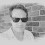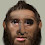Believe those who are seeking the truth. Doubt those who find it. Andre Gide

## Thursday, September 5, 2013

### NGDP targeting and the Taylor Rule

Chris Waller pointed out to me this morning that the NGDP target is formally equivalent to a special case of the Taylor rule. (Maybe this is generally known? I don't know.)

The argument goes as follows. Let R denote the nominal interest rate. Then the NGDP target proposes a monetary policy rule of the form:

R = R* + [log(NGDP) - log(NGDP*)]

where the starred variables denote targets.  So the rule above raises the interest rate when NGDP is above target and lowers the interest rate when NGDP is below target.

Of course, NGDP = PY, so log(NGDP) = log(P) + log(Y).

Thus, we may rewrite our policy rule in the following way:

R = R* + [log(P) +log(Y) - log(P*) - log(Y*)]

Now add and subract the lagged value of log(P) from the RHS of the equation above to get:

R = R* + [log(P) - log(P-) +log(Y) - log(P*) + log(P-) - log(Y*)]

or

R = R* + A*[log(P/P-) - log(P*/P-)]  + B*[log(Y) - log(Y*)]

So the NGDP targeting rule proposes to adjust the nominal interest rate in terms of the prevailing inflation and output gaps, with weights A = B = 1.

It seems surprising that the solution A=B=1 is generically robust. But maybe it is. In many models, A>1 is required for stability. This is the so-called Taylor principle. It is also a property of learning models; see Howitt 1992.

So maybe the NGDP targeting crowd is just saying that A should be lowered and B increased? Is that it? Have to rush off to a meeting...

1.David,

I think this is well known, but then again it might just be well known to me.

1. If A=B=1 this would satisfy the conditions for a unique equilibrium in a New Keynesian model (see Gali's short textbook on this).

2. The advantage that a nominal income target would conceivably have over a Taylor Rule is that with a nominal income target, you don't have to have knowledge and/or an estimate of the output gap.

1.Leaving aside the issue of growth rates vs. levels, not worrying about potential output estimates is important.

Still, political economy issues are important to most Market Monetarists.

Is all of this left to the macroeconomic experts who come up with the weights? Or are we targeting total spending on output?

Remember, the voters are in charge of all of this in the final analysis. What can they understand? Or do we just tell them it is beyond them and trust the experts?

Even if you go one level up to the politicians, it is little better.

2.In the model above, the introduction of potential output was simply incorrect.

Nominal GDP targeting says nothing about potential output.

Level targeting means the log P + log y = constant.

log Pt - log Pt-1 = -(log y - log yt-1)

If real output starts at potential and falls 2% below, and then resumes growing at the same rate as potential, and the inflation rate rises 2% and then goes back to its initial value, then nominal GDP remains on target and the output gap is positive permanently. There is no policy response.

The output gap has no impact on monetary policy.

Not a Taylor rule.

Of course, an output gap might not be consistent with constant inflation. If inflation slows and the output gap doesn't fall, then nominal GDP must be falling below target and there would be a policy response.

2.David Andolfatto:
"Chris Waller pointed out to me this morning that the NGDP target is formally equivalent to a special case of the Taylor rule. (Maybe this is generally known? I don't know.)"

http://www.dallasfed.org/assets/documents/research/staff/staff1202.pdf

All in the Family: The Close Connection Between Nominal-GDP Targeting and the Taylor Rule
Evan F. Koenig
March 2012

Abstract:
"The classic Taylor rule for adjusting the stance of monetary policy is formally a special case of nominal- gross-domestic-product (GDP) targeting. Suitably implemented, moreover, nominal-GDP targeting satisfies the definition of a "flexible inflation targeting" policy rule. However, nominal-GDP targeting would require more discipline from policymakers than some analysts think is realistic."

It's the other way around. The Taylor rule is the special case of NGDP targeting (pages 7-11):

"Note that the Taylor rule is a special case of nominal-GDP targeting... The chief difference between the two policy approaches is that under nominal-GDP targeting, policymakers look at a longer history of price changes than they do under the Taylor rule when deciding on the appropriate policy setting. Secondarily, the estimate of potential output that enters the nominal-GDP-targeting rule is less sensitive to short-term supply shocks than is the estimate that enters the Taylor rule."

David Andolfatto:
"It seems surprising that the solution A=B=1 is generically robust. But maybe it is. In many models, A>1 is required for stability. This is the so-called Taylor principle. It is also a property of learning models; see Howitt 1992."

The Taylor Principle only applies when you are targeting the inflation rate, not when you are targeting the price level.

As the above paper notes:

Introduction:
"Nominal-GDP targeting does imply tighter control of infl‡ation expectations than does the Taylor rule, however, so a shift to nominal-GDP targeting would commit Fed officials to more disciplined policymaking."

Conclusion:
"Consequently, nominal-GDP targeting is less forgiving of near term expected deviations of infl‡ation from long-run target infl‡ation than is the Taylor rule: It requires that any near-term expected in‡flation shortfall be o¤set by expected forward infl‡ation overages so that expected average infl‡ation over the medium term holds steady."

1.Somebody sent me the Koenig paper already (was it you?). I'll give it a read this weekend. Thanks, Mark.

3.Most of them don't think in terms of interest rates, and emphasize that rates drop when people expect poor growth and rise on expectations of high growth. So they'd actually like to cause rates to rise during a recession as a result of raising growth expectations.

1.In the model above, there is R*.

Market Monetarists would not include an R*. There would be no notion that the nominal interest rate can only change if nominal GDP is way from target.

4.I argued a couple of years ago that NGDP targeting would be a better Taylor Rule than the ones typically applied. (Of course most of my arguments fall apart if you don't believe in nominal rigidities.)

1.2.Actually, I should credit Steve Williamson with pointing out (in some blog post that I don't remember, but some time prior to mine) that NGDP targeting was a special case of the Taylor rule (probably not the first to do so but the first that I read). Of course he's critical of the Taylor rule in general, but that's where I got the idea.

5."So maybe the NGDP targeting crowd is just saying that A should be lowered and B increased?"

I think, for the most part, they are saying that both A and B, but especially B, should be increased (as I said explicitly in the post linked above and explained why). If I understand this correctly, strict NGDP targeting requires not A=B=1 but A=B=infinity. In practice, "infinity" means "as high as you can take them before the instrument instability problem becomes too severe to justify pursuing the target more aggressively." The general requirement is that A=B. The absolute size of A and B determines how much you react to deviations from target, which presumably could be a matter of differing opinion among NGDP targeting advocates (but I think they would agree the absolute size should be bigger than 1, in order to fulfill unambiguously the stability condition contained in the Taylor principle).

1.Not quite.

The infinity claim does capture that zero weight that Market Monetarists put on interest rate smoothing.

On the other hand, we do not favor infinite changes in the quantity of money or interest rates if nominal GDP deviated from target last period.

Usually, it is expected nominal GDP one year out.

6.As Mr. Harless explained, if you multiply your first policy rule by Z, as in "R = R* + Z [log(NGDP) - log(NGDP*)]", it means that A = B = Z. So, Z can (should) be higher than 1 and actually near of infinity.

7.You forgot about the NGDP targeting magic dust. That is what makes the difference!

1.The magic dust is the simplicity that makes it easier to monitor, thereby solving the policy communication problem.

8.These comments are ignoring the level/rate debate.

Market Monetarists favor targeting the growth path of nominal GDP. The Taylor rule targets the inflation rate.

This equivalence argument would apply better for McCallum, who favors targeting the growth rate of nominal GDP.

My view (which is common among Market Monetarists) is:

Nominal GDP targeting best (least bad)

Inflation targeting bad for aggregate demand shocks, least bad for aggregate supply shocks.

Price level targeting good for aggregate demand shocks, really bad for aggregate supply shocks.

Having an omniscient and benevolent despot run an ad hoc policy to maximize utility is not feasible. Not such despots exist.

9.Nominal GDP level targeting would have a policy rule of the from

R = R* + (NGDP - NGDP*)

NGDP - NGDP* does not equal log NGDP - log NGDP*

Suppose nominal GDP has a target growth rate of 5%. It rises 7% one period and then returns to 5%. Nominal GDP is above the target growth path by approximately 2%. Log NGDP = log NGDP*, but NGDP - NGDP* = .02.

Nominal GDP level targeting is not the same thing as a Taylor rule.

Also the A has to be greater than 1 result comes from breaking down R* to r* plus inflation. That makes R change with inflation by 1+ A. So all we need is A greater than zero.

If r* = 2, then you end up with 1 + 1.5 log(Pt - Pt-1) + .5 (yt - y*t-1)

Whatever you make r*, it just impacts the intercept.

10.David,

I thought I might add that the relation between nominal GDP targeting and the Taylor rule is in the Hall and Papell (2005)intermediate macro text, page 481 - 483. It doesn't require much of a change in the coefficients of the Taylor rule to make the two equivalent.

David Papell

1.Thanks, David.